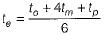Courses

# Test: CPM & PERT - 2

## 10 Questions MCQ Test Topicwise Question Bank for Mechanical Engineering | Test: CPM & PERT - 2

Description
This mock test of Test: CPM & PERT - 2 for Mechanical Engineering helps you for every Mechanical Engineering entrance exam. This contains 10 Multiple Choice Questions for Mechanical Engineering Test: CPM & PERT - 2 (mcq) to study with solutions a complete question bank. The solved questions answers in this Test: CPM & PERT - 2 quiz give you a good mix of easy questions and tough questions. Mechanical Engineering students definitely take this Test: CPM & PERT - 2 exercise for a better result in the exam. You can find other Test: CPM & PERT - 2 extra questions, long questions & short questions for Mechanical Engineering on EduRev as well by searching above.
QUESTION: 1

Solution:
QUESTION: 2

### In PERT, the distribution of activity times is assumed to be

Solution:

In PERT the activity times follow Beta distribution.

QUESTION: 3

### In PERT analysis a critical activity has

Solution:
QUESTION: 4

The expected time (te) of a PERT activity in term s of optimistic time (to), pessimistic time (tp) and most likely time (tm) is given by

Solution:

The expected time (te) of PERT activity in terms of optimistic time (to), pessimistic-time (tp) and most likely time (tm ) is given by,QUESTION: 5

The critical path of a network is the path that

Solution:
QUESTION: 6

In PERT the span of time between the optimistic and pessimistic time estimates of an activity is

Solution:
QUESTION: 7

In an activity has zero slack, it implies that

Solution:
QUESTION: 8

Activity-on-Arrow (AOA) diagram is preferred over Activity-on-Node (AON) diagram because

Solution:
QUESTION: 9

The most likely time (m) is ’mode’ of the

Solution:
QUESTION: 10

The variance of the completion time for a project is the sum of variance of

Solution: